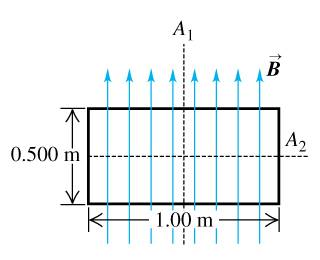# Moment of Inertia of a uniform rectangular coil

dpeagler

## Homework Statement

A uniform rectangular coil of total mass 270 g and dimensions 1m x 5m is oriented perpendicular to a uniform 4.00-T magnetic field (the figure ). A current of 2.90 A is suddenly started in the coil.

## Homework Equations

angular acceleration = torgue / moment of inertia

## The Attempt at a Solution

Here is the image...

It rotates around axis 2I can't figure out how to find the moment of inertia of this shape. I know the basic formula, of moment of inertia and how you find it, but can't figure out how to tell how the mass is distributed. I'm pretty sure the parts of the rectangle that are parallel to the axis of rotation can be excluded, because they will cancel each other out, but the bars on the side are confusing me.

Any help is greatly appreciated.